# Analyze a Subband of Input Frequencies Using Zoom FFT

The `Zoom FFT` block implements zoom FFT based on the multirate multistage bandpass filter designed in Complex Bandpass Filter Design. If you specify the center frequency and the decimation factor, the `Zoom FFT` block designs and applies the filter to the input signal. Using zoom FFT, you can zoom into the tones of the input sine wave.

The input is a noisy sine wave signal with frequencies at 1 kHz and 1.4 kHz. The noise is an additive white Gaussian noise with zero mean and a variance of 1e-2. The input sample rate, Fs, is 44.1 kHz and the input frame size, L, is 440 samples.

Configure the `Zoom FFT` block to analyze a bandwidth of 800 Hz with the center frequency at 1200 Hz. The decimation factor, D, is the ratio of the input sample rate, 44.1 kHz, and the bandwidth of interest, 800 Hz. The FFT length is the ratio of input frame size, 440, and the decimation factor. The FFT is computed over frequencies starting at 800 Hz and spaced by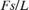Hz apart, which is the resolution or the minimum frequency that can be discriminated. With the above values, the resolution is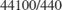, or approximately 100 Hz.

Open the model.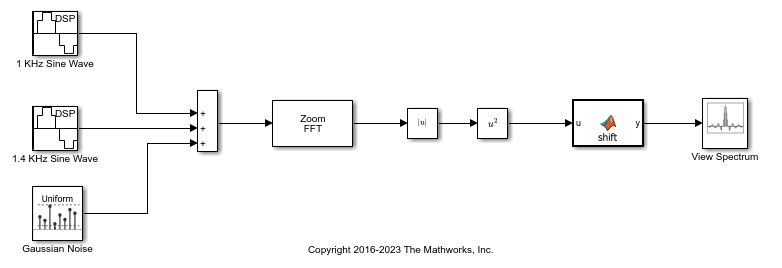Run the model. Compute the square of the magnitude of the zoom FFT output, perform FFT shift, and view the resulting spectrum in array plot.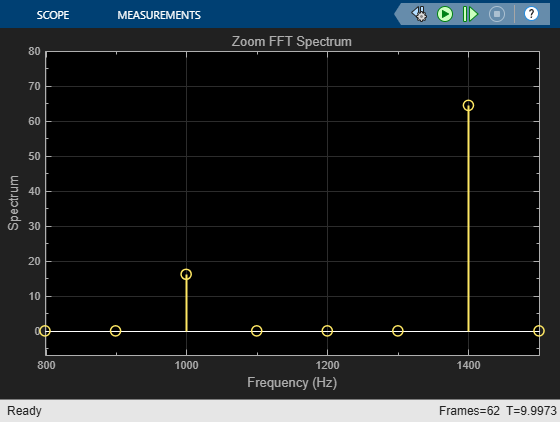The spectrum shows the frequencies in the range [800 1600] Hz, with tones at 1 kHz and 1.4 kHz. The FFT length reduced to length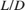. This is the basic concept of zoom FFT. By decimating the original signal, you can retain the same resolution you would achieve with a full size FFT on your original signal by computing a small FFT on a shorter signal. You can alternatively achieve a better resolution by using the same FFT length.

If you make any changes to the model, save the model before closing the model.